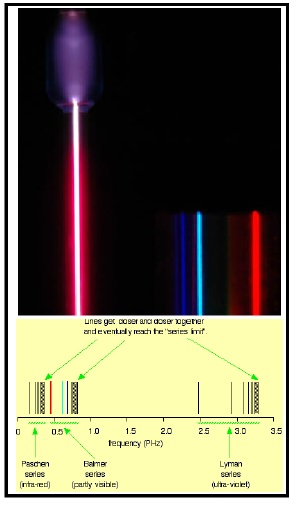### ACM 30210 Foundations of Quantum MechanicsI do not currently teach this module. The materials on this page are outdated and are left here for archival purposes only.

Description: This module introduces Quantum Mechanics in its modern mathematical setting. Several canonical, exactly-solvable models are studied, including one-dimensional piecewise constant potentials, Dirac potentials, the harmonic oscillator, and the Hydrogen atom. Three calculational techniques are introduced: time-independent perturbation theory, variational methods, and numerical (spectral) methods.

Topics and techniques discussed include but are not limited to the following list:

• The postulates of Quantum Mechanics
• Mathematical background: Complex vector spaces and scalar products, linear forms and duality, the natural scalar product derived from linear forms, Hilbert spaces, linear operators, commutation relations, expectation values, uncertainty
• Time evolution and the Schrodinger equation: Derivation of the Schrodinger equation for time-independent Hamiltonians, the position and momentum representations, the probability current, the free particle
• Piecewise constant one-dimensional potentials: Bound and unbound states, wells and barriers, scattering, transmission coefficients, tunneling
• The harmonic oscillator: Solution by power series, Hermite polynomials, creation and annihilation operators, coherent states
• The Hydrogen atom: Solution by separation of variables, quantization of energy and angular momentum, general treatment of central potentials in terms of spherical harmonics
• Angular momentum: Motivation: angular momentum in the hydrogen atom, as derived from spherical harmonics, angular momentum in the abstract setting, intrinsic angular momentum, addition of angular momenta, Clebsch-Gordan coefficients
• Approximation methods: Time-independent perturbation theory: the non-degenerate case, variational methods for estimating the ground-state energy
• Further topics may include Spin coherent states, how to build a microwave laser, the Dyson series for time-evolution for time-dependent Hamiltonians, one-dimensional Dirac potentials, time-independent perturbation theory for degenerate eigenstates, the fine structure of Hydrogen, numerical (spectral) methods for solving the Schrodinger equation

Learning Outcomes: On completion of this module students should be able to:

1. Perform standard linear-algebra calculations as they relate to the mathematical foundations of Quantum Mechanics
2. Solve standard problems for systems with finite-dimensional Hilbert spaces, e.g. the two-level system
3. Solve standard one-dimensional models including piecewise constant potential wells and barriers, Dirac potentials, and the Harmonic oscillator
4. Perform calculations based on Hermite polynomials, including the characterization of coherent states
5. Compute expectation values for appropriate observables for the Hydrogen atom
6. Explain the quantum theory of angular momentum and compute expectation values for appropriate observables. These computations will involve both the matrix representation of intrinsic angular momentum, and the spherical-harmonic representation of orbital angular momentum
7. Add independent angular momenta in the quantum-mechanical fashion
8. Perform time-independent non-degenerate perturbation theory up to and including the second order

### Module Documents

This module will be taught by blended learning in 2020-2021. This means a lot more reliance on online technologies. Therefore, the module materials will regretabbly be put behind a paywall, sorry, available only on Brightspace, for the academic year 2020-2021. However, an old version of the lecture notes (from 2019) is made available here as a resource.